# Huffman code

Jump to: navigation, search

An algorithm for the construction of optimal (i.e., minimum redundancy) variable-length-ary codes () for finite memoryless information sources with known statistics was proposed by D. Huffman in 1952 (see [a1], [a2]). (The algorithm refines the Morse alphabet, as it associates the shortest codewords to the most probable source letters.)

Let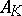be such a source withletters,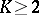, and let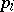be the probability that the source emits letter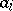(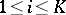). Let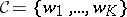be a uniquely decipherable-ary code for(i.e., a set ofsequencesfrom an alphabet ofsymbols) and letbe the length (or number of-ary symbols) of codeword() associated to the letter. The differencebetween the average lengthof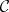and the source entropy (cf. also Entropy)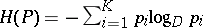is called the redundancy of. A Huffman code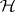forminimizes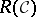(hence) over the set of all uniquely decipherable codes for.

In the binary case (), the algorithm consists in the construction of a sequence of "reduced" sources, where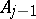is obtained from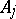by merging the two least probable letters ofinto one letter of(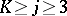) and leaving all other letters unchanged (ties are broken arbitrarily). The probability of the new letter is the sum of the probabilities of the merged letters. Themergings can be represented by a binary code tree in which each letter ofcorresponds to a leaf and each codeword is the sequence of binary labels of the path from root to leaf. (If the code alphabet has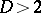letters, each reduced source is obtained by merging theleast probable letters of the previous source, except possibly in the first step, whereletters have to be grouped together,being the remainder of the division ofby. The resulting-ary code tree is labeled and again the codewords are the sequences of labels from root to leaves.)

Owing to the arbitrary resolution of possible ties in grouping the least probable sequences at each step, for a given sourcethere may be several distinct Huffman codes, but two such codes are considered (essentially) different only if the corresponding sets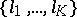of codeword lengths do not coincide. However, the average length of all Huffman codes foris the same.

More formally, consider the set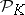of all-order probability distributions (cf. Probability distribution)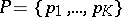,,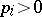(). The informational divergence (also called the Kullback–Leibler information) between two probability distributions,inis the non-negative quantity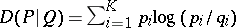.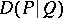vanishes if and only if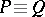, hence it can be considered as an oriented pseudo-distance (cf. also Pseudo-metric) in. Now, ifis the set of codeword lengths of a Huffman codefor, the redundancy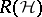is equal to the informational divergence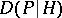between the source probability distributionand the "dyadic" probability distribution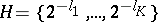.

The optimality of Huffman codes can now be restated by saying that to any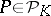(i.e., to any source), the algorithm associates (one of) the dyadic probability distribution(s) "closest" toin the sense of the pseudo-metric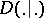.

In several practical situations the statistics of the source is not perfectly known or varies over time, and the necessity arises of updating the Huffman code when the new(ly known)becomes "closer" to a dyadic probability distribution different from the previous one (see [a3], [a4]).

See also Coding and decoding; Information theory.